Updating search results...

# 31 Results

View
Selected filters:
• NC.Math.8.EE.8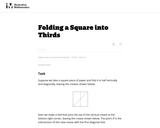Unrestricted Use
CC BY
Rating
0.0 stars

This is a task from the Illustrative Mathematics website that is one part of a complete illustration of the standard to which it is aligned. Each task has at least one solution and some commentary that addresses important asects of the task and its potential use. Here are the first few lines of the commentary for this task: Suppose we take a square piece of paper and fold it in half vertically and diagonally, leaving the creases shown below: Next we make a fold that joins ...

Subject:
Mathematics
Material Type:
Activity/Lab
Provider:
Illustrative Mathematics
Provider Set:
Illustrative Mathematics
Author:
Illustrative Mathematics
09/04/2013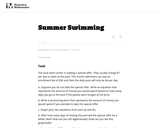Unrestricted Use
CC BY
Rating
0.0 stars

This is a task from the Illustrative Mathematics website that is one part of a complete illustration of the standard to which it is aligned. Each task has at least one solution and some commentary that addresses important asects of the task and its potential use. Here are the first few lines of the commentary for this task: The local swim center is making a special offer. They usually charge \\$7 per day to swim at the pool. This month swimmers can pay an enrollment fee of ...

Subject:
Mathematics
Material Type:
Activity/Lab
Provider:
Illustrative Mathematics
Provider Set:
Illustrative Mathematics
Author:
Illustrative Mathematics
05/15/2013Conditional Remix & Share Permitted
CC BY-NC-SA
Rating
0.0 stars

This 8th grade Math&nbsp;parent guide&nbsp;explains the content in straightforward terms so they can support their children&rsquo;s learning at home and will&nbsp;encourage&nbsp;caretaker engagement&nbsp;with lessons.

Subject:
Mathematics
Material Type:
Reference Material
Author:
Kelly Rawlston
Letoria Lewis
01/10/2022Conditional Remix & Share Permitted
CC BY-NC-SA
Rating
0.0 stars

Our Teacher Guides are meant to support the use of our online course and unit content. Please use these to accompany the use of our content and for ideas to support struggling learners, those needing extension and for additional resources.

Subject:
Mathematics
Material Type:
Curriculum
Teaching/Learning Strategy
Author:
Kelly Rawlston
Letoria Lewis
05/16/2022Conditional Remix & Share Permitted
CC BY-NC
Rating
0.0 stars

In CK-12 Middle School Math Concepts Grade 8, the learning content is divided into concepts. Each concept is complete and whole providing focused learning on an indicated objective. Theme-based concepts provide students with experiences that integrate the content of each concept. Students are given opportunities to practice the skills of each concept through real-world situations, examples, guided practice and explore more practice. There are also video links provided to give students an audio/visual way of connecting with the content.

Subject:
Mathematics
Material Type:
Full Course
Lesson Plan
Textbook
Unit of Study
Provider:
CK-12 Foundation
Provider Set:
CK-12 FlexBook
12/06/2018Unrestricted Use
CC BY
Rating
0.0 stars

This task presents a real-world problem requiring the students to write linear equations to model different cell phone plans. Looking at the graphs of the lines in the context of the cell phone plans allows the students to connect the meaning of the intersection points of two lines with the simultaneous solution of two linear equations.

Subject:
Mathematics
Material Type:
Activity/Lab
Provider:
Illustrative Mathematics
Provider Set:
Illustrative Mathematics
Author:
Illustrative Mathematics
05/01/2012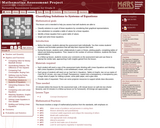Only Sharing Permitted
CC BY-NC-ND
Rating
0.0 stars

This lesson unit is intended to help teachers assess how well students are able to classify solutions to a pair of linear equations by considering their graphical representations. In particular, this unit aims to help teachers identify and assist students who have difficulties in: using substitution to complete a table of values for a linear equation; identifying a linear equation from a given table of values; and graphing and solving linear equations.

Subject:
Algebra
Mathematics
Material Type:
Assessment
Lesson Plan
Provider:
Shell Center for Mathematical Education
Provider Set:
Mathematics Assessment Project (MAP)
06/24/2019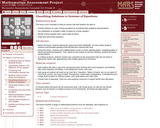Only Sharing Permitted
CC BY-NC-ND
Rating
0.0 stars

This lesson unit is intended to help you assess how well students are able to:

* Classify solutions to a pair of linear equations by considering their graphical representations.
* Use substitution to complete a table of values for a linear equation.
* Identify a linear equation from a given table of values.
* Graph and solve linear equations.

Subject:
Mathematics
Material Type:
Activity/Lab
Formative Assessment
Author:
Mathematics Assessment Resource Service
MARS
08/11/2019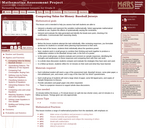Only Sharing Permitted
CC BY-NC-ND
Rating
0.0 stars

This lesson unit is intended to help you assess how well students are able to:

* Interpret a situation and represent the variables mathematically.
* Select appropriate mathematical methods to use.
* Explore the effects of systematically varying the constraints.
* Interpret and evaluate the data generated and identify the break-even point, checking it for confirmation.
* Communicate their reasoning clearly.

Subject:
Mathematics
Material Type:
Activity/Lab
Assessment
Author:
Mathematics Assessment Resource Service
MARS
08/11/2019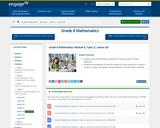Conditional Remix & Share Permitted
CC BY-NC-SA
Rating
0.0 stars

In this lesson, students learn the elimination method for solving a system of linear equations. Students use properties of rational numbers to find a solution to a system, if it exists, through computation using substitution and elimination methods.

Subject:
Mathematics
Material Type:
Lesson Plan
Provider:
EngageNY
02/20/2017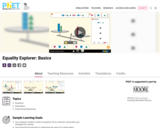Unrestricted Use
CC BY
Rating
0.0 stars

Sample Learning Goals
Use a balance model to solve an equation for an unknown, and justify your strategies for solving
Use proportional reasoning to determine the value of a single object

Subject:
Mathematics
Material Type:
Activity/Lab
Author:
PhET Interactive Simulations
10/05/2021Unrestricted Use
CC BY
Rating
0.0 stars

Sample Learning Goals
Solve and manipulate algebraic equations by applying properties of real numbers (in particular, the inverse properties of addition and multiplication) and properties of equality
Solve and manipulate algebraic equations by substituting different values for a variable
Use a balance model to solve an equation for an unknown, and justify your strategies for solving
Solve an equation using only universal operations

Subject:
Mathematics
Material Type:
Activity/Lab
Author:
PhET Interactive Simulations
10/05/2021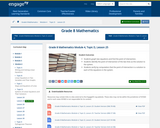Conditional Remix & Share Permitted
CC BY-NC-SA
Rating
0.0 stars

In this lesson, students graph two equations and find the point of intersection. Students identify the point of intersection of the two lines as the solution to the system. Students verify by computation that the point of intersection is a solution to each of the equations in the system.

Subject:
Mathematics
Material Type:
Lesson Plan
Provider:
EngageNY
02/20/2017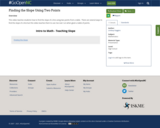Unrestricted Use
CC BY
Rating
0.0 stars

This video teaches students how to find the slope of a line using two points from a table.&nbsp; There are several ways to find the slope of a line but this video teaches them to use rise over run when given a table of points.

Subject:
Mathematics
Material Type:
Presentation
Author:
Lindsay Goggins
08/16/2021Unrestricted Use
CC BY
Rating
0.0 stars

This task can be used to both assess student understanding of systems of linear equations or to promote discussion and student thinking that would allow for a stronger solidification of these concepts.

Subject:
Mathematics
Material Type:
Activity/Lab
Provider:
Illustrative Mathematics
Provider Set:
Illustrative Mathematics
Author:
Illustrative Mathematics
05/01/2012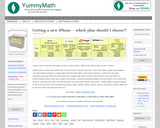Rating
0.0 stars

For this activity, students select a cellular plan considering the cost of the phone and plan over time. Students create tables, graphs, and equations and compare paying for the phone up front or with installment payments using popular cellular companies.

Subject:
Mathematics
Material Type:
Interactive
Provider:
YummyMath
04/26/2017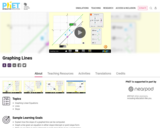Unrestricted Use
CC BY
Rating
0.0 stars

Sample Learning Goals
Explain how the slope of a graphed line can be computed.
Graph a line given an equation in either slope-intercept or point-slope form.
Write an equation in slope-intercept or point-slope form given a graphed line.
Predict how changing variables in a linear equation will affect the graphed line.

Subject:
Mathematics
Material Type:
Activity/Lab
Author:
PhET Interactive Simulations
10/05/2021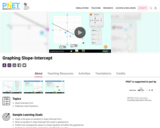Unrestricted Use
CC BY
Rating
0.0 stars

Sample Learning Goals
Graph a line given an equation in slope-intercept form.
Write an equation in slope-intercept form given a graphed line.
Predict how changing the values in a linear equation will affect the graphed line.
Predict how changing the graphed line will affect the equation.

Subject:
Mathematics
Material Type:
Activity/Lab
Author:
PhET Interactive Simulations
10/05/2021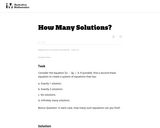Unrestricted Use
CC BY
Rating
0.0 stars

This task requires students to find linear equations with different numbers of solutions.

Subject:
Mathematics
Material Type:
Activity/Lab
Provider:
Illustrative Mathematics
Provider Set:
Illustrative Mathematics
Author:
Illustrative Mathematics
05/01/2012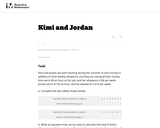Unrestricted Use
CC BY
Rating
0.0 stars

In this task students use linear equations to solve a real world problem.

Subject:
Mathematics
Material Type:
Activity/Lab
Provider:
Illustrative Mathematics
Provider Set:
Illustrative Mathematics
Author:
Illustrative Mathematics